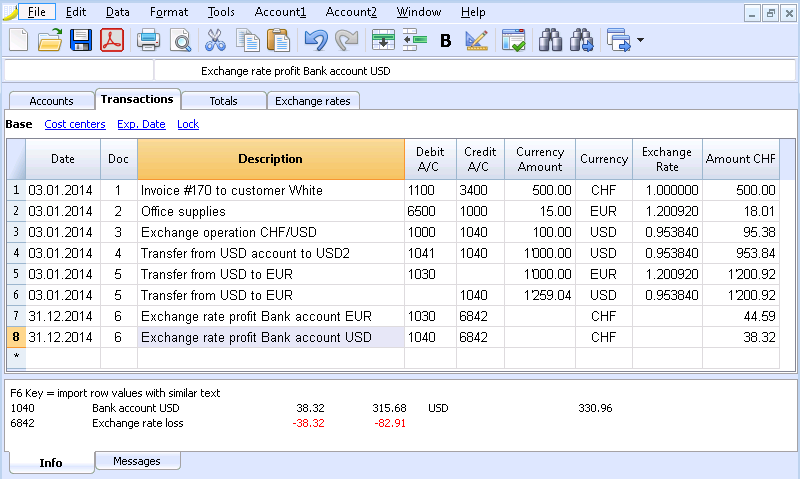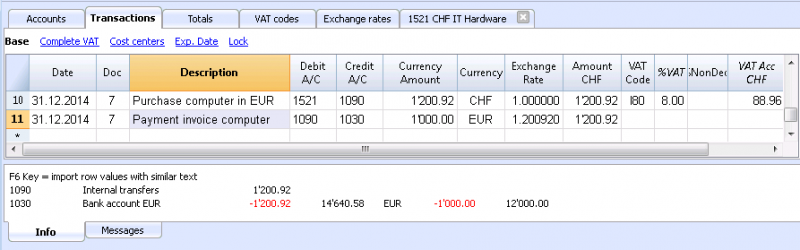# Transactions

#### This documentation is outdated

The most complete and up-to-date documentation is the one of Banana Accounting Plus: Try it now

With regard to the exchange rates, multiplier and historical exchange rates, please refer to the page "Exchange rates table"

## Explanations of the columns

In the Transactions table of the Multi-currency accounting there are the following extra columns:

• Currency amount
This is the amount of the currency specified in the column with the currency symbol.
This amount is used by the program to update the balance of the related account in currency.
• Currency
This is the currency symbol of the currency to which the amount refers.
The currency symbol has to be the Basic currency, specified in the File and accounting properties, or the currency symbol of an annount indicated in the columns  Debit A/C or Credit A/C.
You can also use a different currency as long as the indicated Debit A/C and the Credit A/C are Basic currency accounts.
In this case the amount in currency is used as a reference, but will not be used for accounting purposes
• Exchange rate
Used to convert the foreign currency amount in its Basic currency equivalent.
• Amount in Basic currency
The transaction amount, expressed in Basic currency.
This amount is used by the program to update the balance of the related account in Basic currency
• Exchange rate multiplier
Normally not visible in the view, this value is multiplied by the exchange rate.

##For each transaction, there are two accounts (debit account and credit account). In the program, only one foreign curency per transaction row can be used. So there can be the following direct combinations:

• Entries between two accounts in basic currency with the amount in basic currency. (in the image, Doc 1 row)
The account currency is the basic currency.
• Entries between two accounts in basic currency with the amount in foreign currency. (Doc 2)
The indicated accounts are in basic currency, but the currency symbol and the amount in currency, indicated in the transaction row, are not in basic currency, but in a different currency.
To insert the different currency, the user has to manually change the currency symbol.
This is being used when one goes abroad and money is being changed in order to pay in local currency. In this case we do not have a specific account.
For the calculation of the balance (both accounts being in basic currency) only the amount of basic currency column is being used.
• Entries between an account in foreign currency and one in basic currency  (Doc 3)
The currency needs to be the one of the account in foreign currency.
For the calculation of the balance of the account in foreign currency, the program uses the amount in currency and for the balance in basic currency, the program uses the amount in basic currency.
• Entries between two accounts with the same foreign currency (Doc 4)
The currency needs to be the same as account currency of both accounts.
• Entries with two accounts in different foreign currencies (Doc 5)
For example, the bank makes an exchange operation between two foreign currencies:
In this case, the transaction needs to be recorded on two rows.
The amount in basic currency needs to be the same. It is useful to use an amount close to the current exchange rate to avoid excessive exchange rate differences.
In order for the amounts in basic currency to be equal, the amount in basic currency needs to be indicated manually, and the program will calculate the exchange rate.
• Exchange rate differences (Doc 6)
The goal of this transaction is to realign the balance of the Basic currency account with the equivalent of the Foreign currency account at today's exchange rate.
On the Foreign currency account, only the amount in Basic currency related to the exchange rate differences is being recorded.
They are automatically generated with the Create transaction for exchange rate variation command.
• For the exchange rate profits, the account to revaluate is indicated in debit and the exchange rate profits account in credit.
• For the exchange rate losses, the account to revaluate is indicated in credit and the exchange rate losses account in debit.
• The Currency amount is being left empty
• The Curency symbol is the basic currency
• In the Basic currency amount column, the amount of the revaluation of the account (profit or loss) is being indicated.

Establishing the exchange rate

The accountant is the one who decides which exchange rate to use for each single operation. Generally, the following rusles are being applied:

• For normal operations, the exchange rate of the day is being used
• For buying or selling currency, the values indicated by a money exchange office or a bank are being used.
First the amount in foreign currency is being indicated in the program and then the amount in basic currency. The program calculates the exchange rate. The exchange rate indicated by the bank can be slightly different, because banks specify exchange rates with few figures after the decimal point and often round the amounts.
• When several operations with the same exchange rate are being recorded, it is useful to update the exchange rate in the Exchange rate table, so that the program can automatically apply it.
• For operations from abroad that are subject to VAT, the national authority might impose a standard exchange rate. In thit case, that exchange rate should be inserted in the Exchange rate column of the transaction
• To purchase real estate or equity investments, an historical exchange rate is being used. In that case, a currency symbol needs to be created in the Exchange rate table (for example USD1) with an historical exchange rate, that is not being subject to the fluctuations of the exchange rate.
One can create as many currency symbols as desired for all historical exchange rates.

## Transactions with VAT

The VAT account and the account from which the VAT is being deducted have to be in basic currency. It is impossible to use a VAT code tto deduct the VAT from a foreign currency account.

In order to record operations with VAT that have accounts in foreign currency as their counterpart, two transaction rows have to be used:

• First, the amount of the purchase is being recorded on an Internal transfers account in basic currency and the related VAT code is being applied. The amount in basic currency has to be calculated using an exchange rate in accordance with the requirements of the Tax administration.
• In a second row, the balance of the Internal transfers account is being put to zero; as its counterpart, the account in foreign currency should be entered.
The amount used for this transaction, both in basic currency and in foreign currency, has to be excluding VAT. Obviously, the exchange rate that has to be used is the same one as the one being used in the preceding transaction.

In the example, the basic currency is the CHF. We are dealing with a national purchase, but paid from a foreign curency account (EUR).## Automatisms while entering multi-currency transactions

When a new transaction is being entered, the data in the above mentioned columns have to be completed.

When different values of the transaction row are being modified, the program completes the transaction with the predefined values. If these values do not satisfy the user's requirements, these have to be modified in the transaction row.

The modification of the values in the Exchange rate table have no effect on already inserted transaction rows. Thus, when the exchange rate in the Exchange rate table is being modified, this has no influence whatsoever on already inserted transactions.

• When the amount in currency is being entered and there are a Debit A/C and a Credit A/C, and no other values are present
• The currency symbol is being retrieved from the account in use, giving priority to the account that is not in basic currency.
• The exchange rate, defined in the Exchange rate table, is being applied with the following logic:
• The historical exchange rate is being applied, with a date earlier or equal to the transaction date
• If there is no historical exchange rate to be found, the exchange rate from the row without date is being applied.
• The multiplier, defined in the Exchange rate table, is being applied or the number 1 if it is the basic currency.
• The amount in basic currency is being calculated.
• If the amount in currency is being modified (and there are already other values present):
the amount in basic currency is being calculated, with the existing exchange rate
• If the currency symbol is being modified:
the exchange rate with the multiplier is being applied and the amount in basic currency is being calculated (like above)
• If the exchange rate is being modified
The amount in basic currency is being calculated, using the entered exchange rate.
• When the amount in basic currency is being modified:
The exchange rate is being recalculated.

## More help

• While being positioned on the amount in currency column and pressing the F6 key, the program rewrites all values with the earlier explained logic, as if there were no values present. This feature is useful when the Debit A/C or the Credit A/C is being modified
• Smart fill Exchange rate column
The program suggests several exchange rates, picking them up from the Exchange rates table or from exchange rates used earlier in the transactions.

## Info window

In the info window, the program indicates:

• Differences, if any, between the total movements in debit and the total movements in credit  f
• Indications on the subject of the F6 key

For the accounts related to the transaction row on which one is positioned:

• The account number
• The account description
• The amount of the transaction in basic currency
• The current account balance in basic currency
• Currency symbol of the account
• The transaction amount on the account in the currency of the account
• The current account balance in currency

## Data transfer from earlier versions

In version 4 or earlier, the absence of a currency symbol in the Transactions table was being interpreted as a transaction in basic currency.
In version 7, each transaction needs to have its own currency symbol. Therefore, when you update from version 4 to version 7, in the accounting file, the transactions without a currency symbol need to be completed.

help_id
multicurrency::transactions